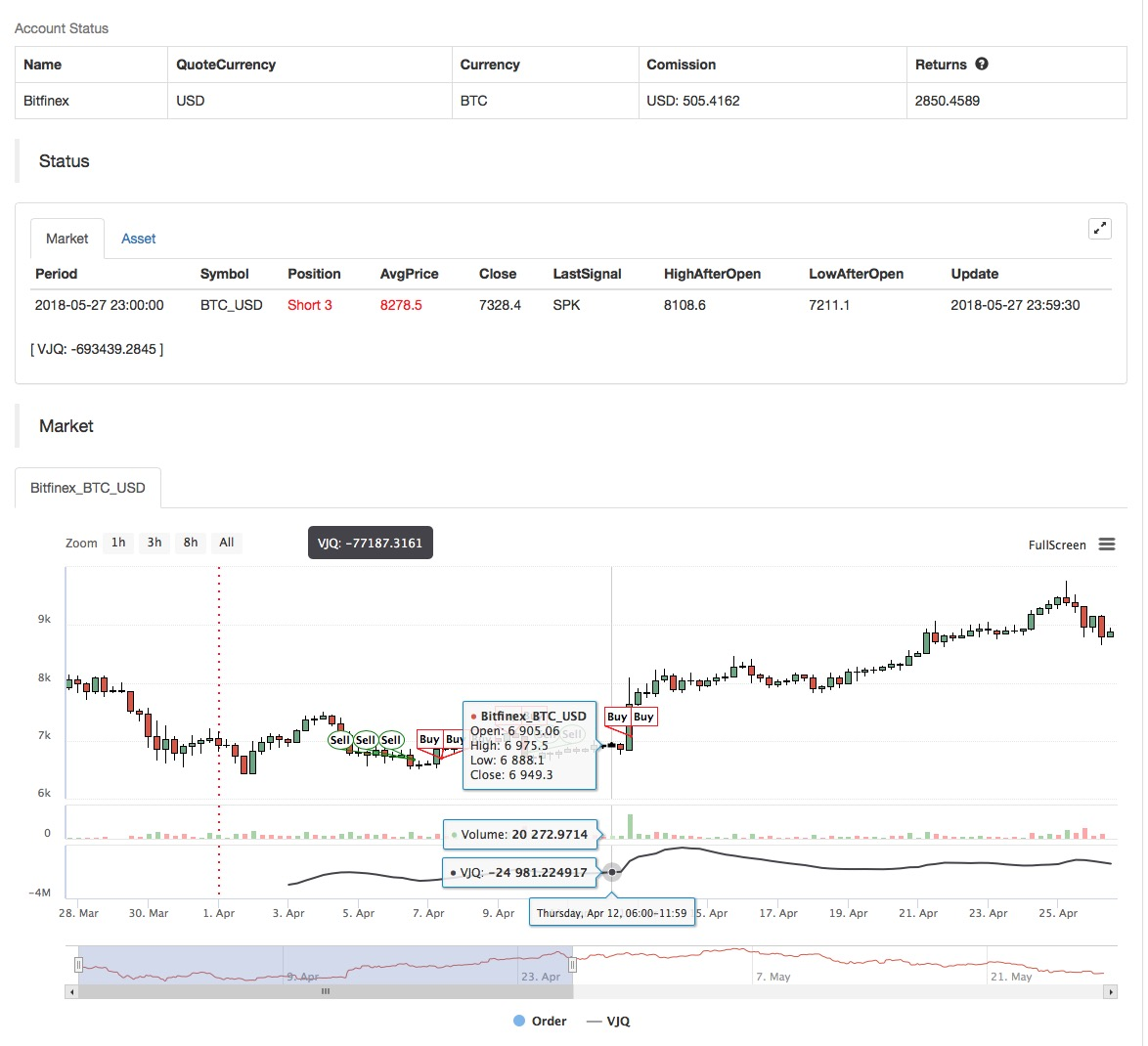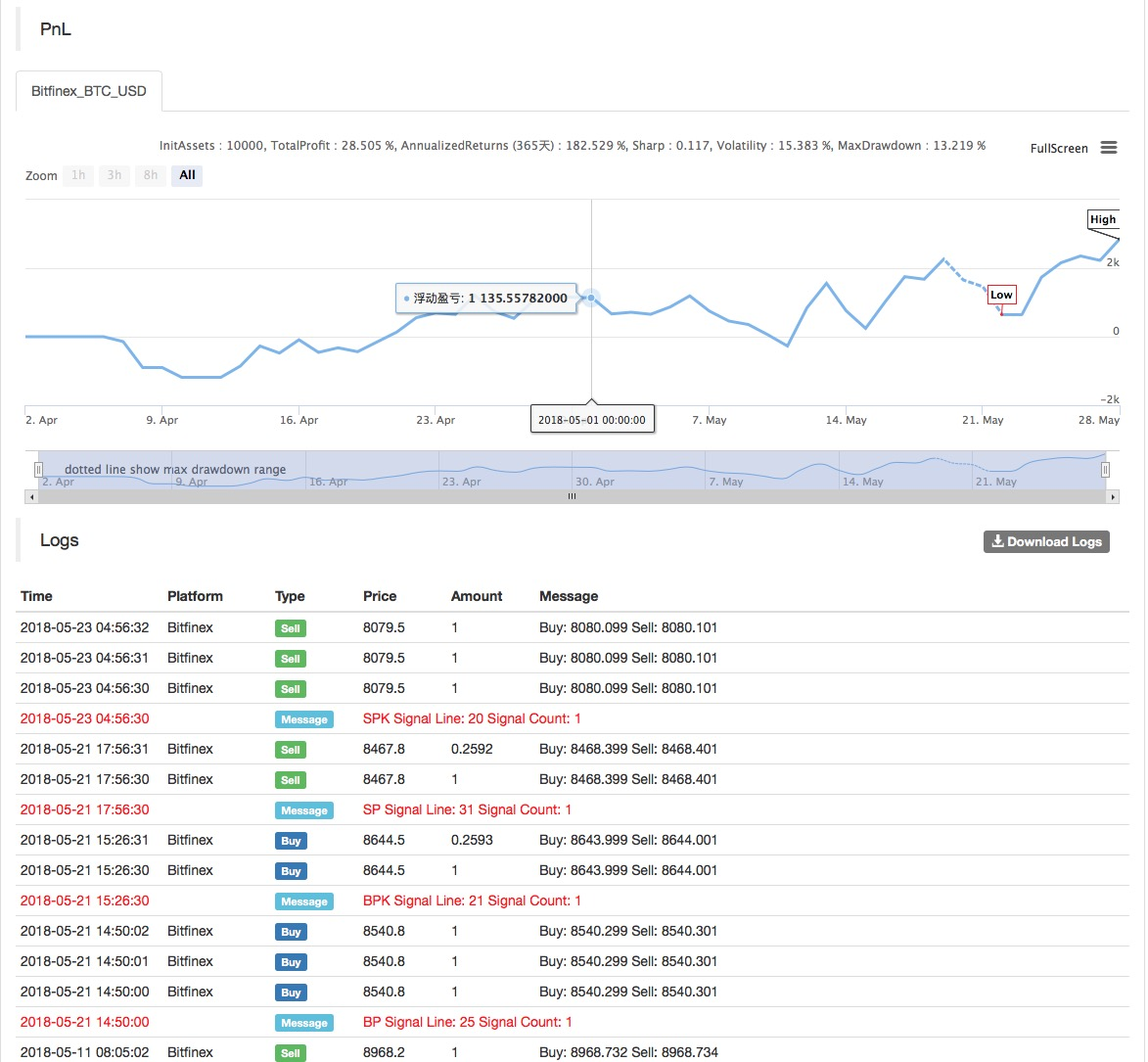# 运用成交量指数加权的量化交易策略

### 成交量指数加权策略在发明者量化平台的运用

• 数据周期：多周期

• 回测标的：OKEX 期货

• 合约类型：this_week``````MAN^^MA(C,N);
B_MA:=C>MAN;
S_MA:=C<MAN;

S_K1:=SUM((H-C)*V,N)/SUM((H-L)*V,N)>0.5;
B_K1:=SUM((C-L)*V,N)/SUM((H-L)*V,N)>0.5;

CO:=IF(C>O,C-O,0);
OC:=IF(C<O,O-C,0);
S_K2:=SUM(OC*V,N)/SUM(ABS(C-O)*V,N)>0.5;
B_K2:=SUM(CO*V,N)/SUM(ABS(C-O)*V,N)>0.5;

B_K1 AND B_K2 AND B_MA AND H>=HHV(H,N),BPK;
S_K1 AND S_K2 AND S_MA AND L<=LLV(L,N),SPK;

STOPLOSS:=M*MA(H-L,N);
C<BKPRICE-STOPLOSS,SP(BKVOL);
C>SKPRICE+STOPLOSS,BP(SKVOL);

S_MA AND BKHIGH>BKPRICE+STOPLOSS,SP(BKVOL);
B_MA AND SKLOW<SKPRICE-STOPLOSS,BP(SKVOL);
``````

##### 相关下载
###### 登录后评论
Copyright © 2019 宽客在线